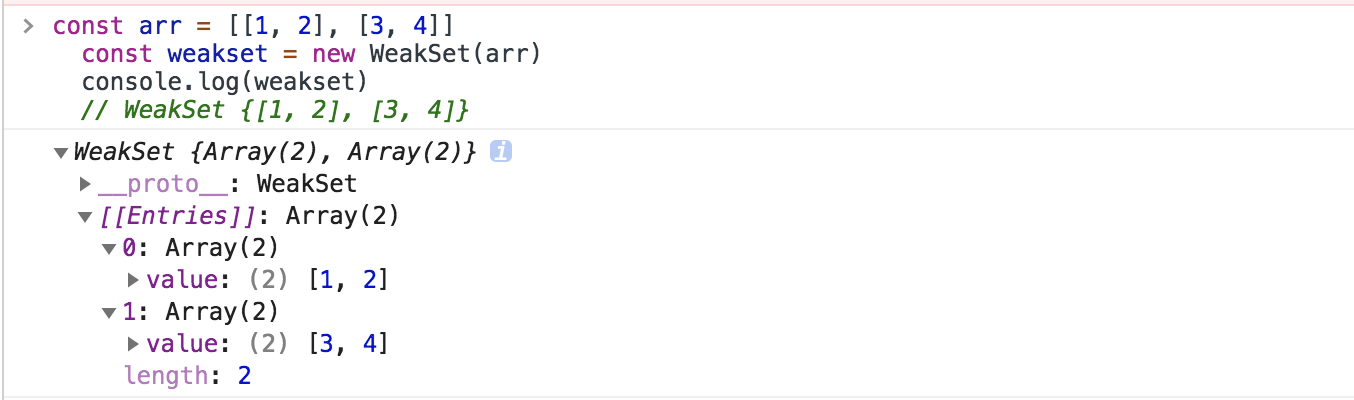# # Set、WeakSet、Map及WeakMap

Set 和 Map 主要的应用场景在于 数据重组数据储存

Set 是一种叫做集合的数据结构，Map 是一种叫做字典的数据结构

## # 1. 集合（Set）

ES6 新增的一种新的数据结构，类似于数组，但成员是唯一且无序的，没有重复的值。

Set 本身是一种构造函数，用来生成 Set 数据结构。

``````new Set([iterable])
``````

``````const s = new Set()
[1, 2, 3, 4, 3, 2, 1].forEach(x => s.add(x))

for (let i of s) {
console.log(i)	// 1 2 3 4
}

// 去重数组的重复对象
let arr = [1, 2, 3, 2, 1, 1]
[... new Set(arr)]	// [1, 2, 3]
``````

Set 对象允许你储存任何类型的唯一值，无论是原始值或者是对象引用。

``````let set = new Set();
let a = NaN;
let b = NaN;
set // Set {NaN}

let set1 = new Set()
console.log([...set1])	// [5, "5"]
``````
• Set 实例属性

• constructor： 构造函数

• size：元素数量

``````let set = new Set([1, 2, 3, 2, 1])

console.log(set.length)	// undefined
console.log(set.size)	// 3
``````
• Set 实例方法

• 操作方法

• delete(value)：存在即删除集合中value

• has(value)：判断集合中是否存在 value

• clear()：清空集合

``````let set = new Set()

set.has(1)	// true
set.has(3)	// false
set.delete(1)
set.has(1)	// false
``````

`Array.from` 方法可以将 Set 结构转为数组

``````const items = new Set([1, 2, 3, 2])
const array = Array.from(items)
console.log(array)	// [1, 2, 3]
// 或
const arr = [...items]
console.log(arr)	// [1, 2, 3]
``````
• 遍历方法（遍历顺序为插入顺序）
• keys()：返回一个包含集合中所有键的迭代器

• values()：返回一个包含集合中所有值得迭代器

• entries()：返回一个包含Set对象中所有元素得键值对迭代器

• forEach(callbackFn, thisArg)：用于对集合成员执行callbackFn操作，如果提供了 thisArg 参数，回调中的this会是这个参数，没有返回值

``````let set = new Set([1, 2, 3])
console.log(set.keys())	// SetIterator {1, 2, 3}
console.log(set.values())	// SetIterator {1, 2, 3}
console.log(set.entries())	// SetIterator {1, 2, 3}

for (let item of set.keys()) {
console.log(item);
}	// 1	2	 3
for (let item of set.entries()) {
console.log(item);
}	// [1, 1]	[2, 2]	[3, 3]

set.forEach((value, key) =>  {
console.log(key + ' : ' + value)
})	// 1 : 1	2 : 2		3 : 3
console.log([...set])	// [1, 2, 3]
``````

Set 可默认遍历，默认迭代器生成函数是 values() 方法

``````Set.prototype[Symbol.iterator] === Set.prototype.values	// true
``````

所以， Set可以使用 map、filter 方法

``````let set = new Set([1, 2, 3])
set = new Set([...set].map(item => item * 2))
console.log([...set])	// [2, 4, 6]

set = new Set([...set].filter(item => (item >= 4)))
console.log([...set])	//[4, 6]
``````

因此，Set 很容易实现交集（Intersect）、并集（Union）、差集（Difference）

``````let set1 = new Set([1, 2, 3])
let set2 = new Set([4, 3, 2])

let intersect = new Set([...set1].filter(value => set2.has(value)))
let union = new Set([...set1, ...set2])
let difference = new Set([...set1].filter(value => !set2.has(value)))

console.log(intersect)	// Set {2, 3}
console.log(union)		// Set {1, 2, 3, 4}
console.log(difference)	// Set {1}
``````

## # 2. WeakSet

WeakSet 对象允许你将弱引用对象储存在一个集合中

WeakSet 与 Set 的区别：

• WeakSet 只能储存对象引用，不能存放值，而 Set 对象都可以
• WeakSet 对象中储存的对象值都是被弱引用的，即垃圾回收机制不考虑 WeakSet 对该对象的应用，如果没有其他的变量或属性引用这个对象值，则这个对象将会被垃圾回收掉（不考虑该对象还存在于 WeakSet 中），所以，WeakSet 对象里有多少个成员元素，取决于垃圾回收机制有没有运行，运行前后成员个数可能不一致，遍历结束之后，有的成员可能取不到了（被垃圾回收了），WeakSet 对象是无法被遍历的（ES6 规定 WeakSet 不可遍历），也没有办法拿到它包含的所有元素

• constructor：构造函数，任何一个具有 Iterable 接口的对象，都可以作参数

``````const arr = [[1, 2], [3, 4]]
const weakset = new WeakSet(arr)
console.log(weakset)
``````• has(value)：判断 WeakSet 对象中是否包含value
• delete(value)：删除元素 value
• clear()：清空所有元素，注意该方法已废弃
``````var ws = new WeakSet()
var obj = {}
var foo = {}

ws.has(window)	// true
ws.has(foo)	// false

ws.delete(window)	// true
ws.has(window)	// false

``````

## # 3. 字典（Map）

• 共同点：集合、字典 可以储存不重复的值
• 不同点：集合 是以 [value, value]的形式储存元素，字典 是以 [key, value] 的形式储存
``````const m = new Map()
const o = {p: 'haha'}
m.set(o, 'content')
m.get(o)	// content

m.has(o)	// true
m.delete(o)	// true
m.has(o)	// false
``````

``````const set = new Set([
['foo', 1],
['bar', 2]
]);
const m1 = new Map(set);
m1.get('foo') // 1

const m2 = new Map([['baz', 3]]);
const m3 = new Map(m2);
m3.get('baz') // 3
``````

``````new Map().get('asfddfsasadf')
// undefined
``````

``````const map = new Map();

map.set(['a'], 555);
map.get(['a']) // undefined
``````

``````let map = new Map();

map.set(-0, 123);
map.get(+0) // 123

map.set(true, 1);
map.set('true', 2);
map.get(true) // 1

map.set(undefined, 3);
map.set(null, 4);
map.get(undefined) // 3

map.set(NaN, 123);
map.get(NaN) // 123
``````

Map 的属性及方法

• constructor：构造函数

• size：返回字典中所包含的元素个数

``````const map = new Map([
['name', 'An'],
['des', 'JS']
]);

map.size // 2
``````

• set(key, value)：向字典中添加新元素
• get(key)：通过键查找特定的数值并返回
• has(key)：判断字典中是否存在键key
• delete(key)：通过键 key 从字典中移除对应的数据
• clear()：将这个字典中的所有元素删除

• keys()：将字典中包含的所有键名以迭代器形式返回
• values()：将字典中包含的所有数值以迭代器形式返回
• entries()：返回所有成员的迭代器
• forEach()：遍历字典的所有成员
``````const map = new Map([
['name', 'An'],
['des', 'JS']
]);
console.log(map.entries())	// MapIterator {"name" => "An", "des" => "JS"}
console.log(map.keys()) // MapIterator {"name", "des"}
``````

Map 结构的默认遍历器接口（`Symbol.iterator`属性），就是`entries`方法。

``````map[Symbol.iterator] === map.entries
// true
``````

Map 结构转为数组结构，比较快速的方法是使用扩展运算符（`...`）。

``````const reporter = {
report: function(key, value) {
console.log("Key: %s, Value: %s", key, value);
}
};

let map = new Map([
['name', 'An'],
['des', 'JS']
])
map.forEach(function(value, key, map) {
this.report(key, value);
}, reporter);
// Key: name, Value: An
// Key: des, Value: JS
``````

1. Map 转 Array

``````const map = new Map([[1, 1], [2, 2], [3, 3]])
console.log([...map])	// [[1, 1], [2, 2], [3, 3]]
``````
2. Array 转 Map

``````const map = new Map([[1, 1], [2, 2], [3, 3]])
console.log(map)	// Map {1 => 1, 2 => 2, 3 => 3}
``````
3. Map 转 Object

因为 Object 的键名都为字符串，而Map 的键名为对象，所以转换的时候会把非字符串键名转换为字符串键名。

``````function mapToObj(map) {
let obj = Object.create(null)
for (let [key, value] of map) {
obj[key] = value
}
return obj
}
const map = new Map().set('name', 'An').set('des', 'JS')
mapToObj(map) // {name: "An", des: "JS"}
``````
4. Object 转 Map

``````function objToMap(obj) {
let map = new Map()
for (let key of Object.keys(obj)) {
map.set(key, obj[key])
}
return map
}

objToMap({'name': 'An', 'des': 'JS'}) // Map {"name" => "An", "des" => "JS"}
``````
5. Map 转 JSON

``````function mapToJson(map) {
return JSON.stringify([...map])
}

let map = new Map().set('name', 'An').set('des', 'JS')
mapToJson(map)	// [["name","An"],["des","JS"]]
``````
6. JSON 转 Map

``````function jsonToStrMap(jsonStr) {
return objToMap(JSON.parse(jsonStr));
}

jsonToStrMap('{"name": "An", "des": "JS"}') // Map {"name" => "An", "des" => "JS"}
``````

## # 4. WeakMap

WeakMap 对象是一组键值对的集合，其中的键是弱引用对象，而值可以是任意

WeakMap 中，每个键对自己所引用对象的引用都是弱引用，在没有其他引用和该键引用同一对象，这个对象将会被垃圾回收（相应的key则变成无效的），所以，WeakMap 的 key 是不可枚举的。

• constructor：构造函数

• has(key)：判断是否有 key 关联对象
• get(key)：返回key关联对象（没有则则返回 undefined）
• set(key)：设置一组key关联对象
• delete(key)：移除 key 的关联对象
``````let myElement = document.getElementById('logo');
let myWeakmap = new WeakMap();

myWeakmap.set(myElement, {timesClicked: 0});

let logoData = myWeakmap.get(myElement);
logoData.timesClicked++;
}, false);
``````

## # 5. 总结

• Set
• 成员唯一、无序且不重复
• [value, value]，键值与键名是一致的（或者说只有键值，没有键名）
• WeakSet
• 成员都是对象
• 成员都是弱引用，可以被垃圾回收机制回收，可以用来保存DOM节点，不容易造成内存泄漏
• Map
• 本质上是键值对的集合，类似集合
• 可以遍历，方法很多可以跟各种数据格式转换
• WeakMap
• 只接受对象作为键名（null除外），不接受其他类型的值作为键名
• 键名是弱引用，键值可以是任意的，键名所指向的对象可以被垃圾回收，此时键名是无效的
• 不能遍历，方法有get、set、has、delete

## # 6. 扩展：Object与Set、Map

1. Object 与 Set

``````// Object
const properties1 = {
'width': 1,
'height': 1
}
console.log(properties1['width']? true: false) // true

// Set
const properties2 = new Set()
console.log(properties2.has('width')) // true
``````
2. Object 与 Map

JS 中的对象（Object），本质上是键值对的集合（hash 结构）

``````const data = {};
const element = document.getElementsByClassName('App');